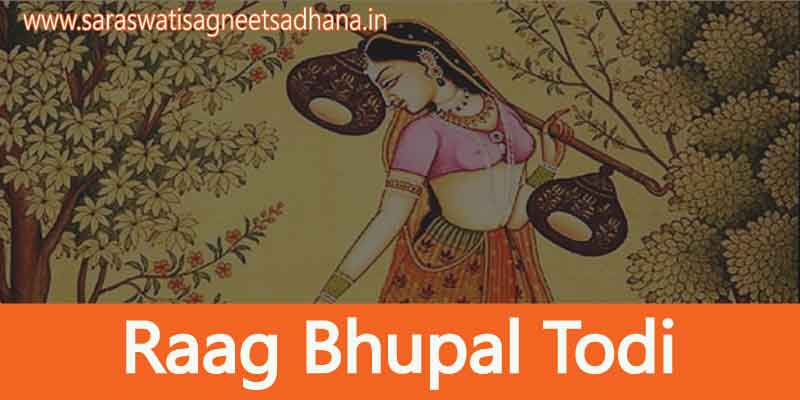# Bhupal Todi Raag Parichay In English Bandish 16 Matras Allap Taan

0
46Bhupal Todi raag is believed to have originated from Bhairavi thaat. In this raag Ga(k)Dha(k) & Re(k) are used as komal swar . Ma &  Ni are not used in this raag ,so its jati is Audav – Audav . In this raag Dha & Ga are Vadi & Samvadi swar .The singing time of this raag is 6 am to 9 am (the first part of the day ).

## Bhupal Todi Raag In English

### How To Read Sargam Notes –

• “.” is used for Mandra saptak(Lower octave notes) swars.eg- .Pa
• “*” is user for Taar Saptak (Higher Octave notes) Swars eg – Pa*
• “(k)” small character is used for komal swars.eg – ( Re(k) , Ga(k) , Dha(k) , Ni(k) )
• Ma(t) here “(t)” is used for showing teevra swar Ma(t) .
• “-” is used for Holdong the notes according to the Song.
• Swars written “ReGa” in this manner means they are playing fast or two swars on one beat.
• Ma(Ga) or (Ga)Ma Here Ga is Kan Swar or Sparsh Swar and Ma is Mool Swar. (You Can skip the kan swar if not understand how to play)
• Swars written [ Ni(k)  Pa ] in this manner they are playing or singing in sliding mode means Meend acc to Indian music .
• { NiSa*Re*Sa* Ni } here this braket {} is used for showing Khatka in which swars are playing fast .

### Description of Raag:-

Aaroh – Sa Re(k) Ga(k) Pa, Dha(k) Sa*.

Avaroh – Sa* Dha(k) Pa, Ga(k) Re(k) Ga(k) Re(k), Sa.

Thaat – Bhairavi thaat

Varjit swar – Ma and Ni

Jati – Audav – Audav(5,5)

Singing time – 6 am to 9 am (the first part of the day ).

### Important Notes:-

1. According to name this is known as type of Todi but this is generated from Bhairav thaat. Because Madhayam is this raag which is important swar of tori raag.

2.This is created to soften swar of bhupali raag.

3.The practice of this raag is similar to raag tori therefore it’s name is Bhupali Todi.

### Swaroop:-

Sa, Re(k) – Re(k) Ga(k) Re(k) Sa, .Dha(k) Sa, .Dha(k) .Pa, .Pa .Dha(k) Sa, Re(k) Ga(k) Re(k) Re(k) Sa, .Dha(k) Sa, .Dha(k) .Re(k) Sa [.Dha(k) Ga(k)] Re(k) Sa|Sa Re(k) Ga(k) Re(k) Ga(k) Pa, Ga(k) Pa Dha(k) Dha(k) Pa, [Dha(k) Ga(k)] – Re(k) Ga(k) Re(k) Sa – Re(k) Ga(k) Pa Dha(k), Pa Dha(k) Ga(k), Ga(k) Pa Dha(k) Ga(k), Re(k) Ga(k) Re(k) Sa, Sa Re(k) Ga(k) Pa Dha(k) Dha(k) Sa*, Dha(k) Dha(k) Sa*, Re(k)* Ga(k) Re(k)* Sa*, Sa* Re(k)* Ga(k)* Re(k)* Sa*, Ga(k)* Re(k)* Sa*, Dha(k), Pa Dha(k) Sa* Dha(k), Dha(k) Pa Ga(k) Pa Ga(k), Re(k) Ga(k) Dha(k) Pa Ga(k), Re(k) Ga(k) Re(k) Sa.

### What are the Aaroh, Avaroh & Pakad of Bhupal Todi raag ?

Aaroh – Sa Re(k) Ga(k) Pa, Dha(k) Sa*.
Avaroh – Sa* Dha(k) Pa, Ga(k) Re(k) Ga(k) Re(k), Sa.

### What is the Jati of Bhupal Todi raag ?

Jati – Audav – Audav(5,5)

### What is the singing time of Bhupal Todi raag ?

Singing time – 6 am to 9 am (the first part of the day).

### Which swars are used in Bhupal Todi raag ?

Aaroh – Sa Re(k) Ga(k) Pa, Dha(k) Sa*.
Avaroh – Sa* Dha(k) Pa, Ga(k) Re(k) Ga(k) Re(k), Sa.

### What is the Thaat of Bhupal Todi raag ?

Thaat – Bhairavi thaat